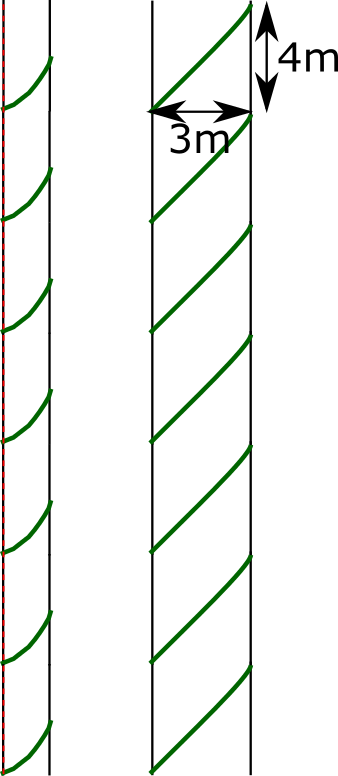#### You may also likeA 1 metre cube has one face on the ground and one face against a wall. A 4 metre ladder leans against the wall and just touches the cube. How high is the top of the ladder above the ground?Four rods are hinged at their ends to form a convex quadrilateral. Investigate the different shapes that the quadrilateral can take. Be patient this problem may be slow to load.### Three Cubes

Can you work out the dimensions of the three cubes?

# Winding Vine

##### Age 14 to 16 Short Challenge Level:
Unwrapping the vine from the pole
Imagine unwrapping the vine from the pole, keeping the top of the vine at the same height as the top of the pole, as shown.Then when the vine is fully unwound, it will form the hypotenuse of a right-angled triangle.The height of the triangle is the same as the height of the pole, 28 metres. The base of the triangle is 7 times the circumference of the pole, 7$\times$3 = 21 metres.

Applying Pythagoras' Theorem, the length of the vine is $\sqrt{28^2+21^2}=35$ metres.

Unwrapping the surface of the pole
Imagine unwrapping the surface of the pole, as shown below, to give a 3 metre wide strip with seven right-angled triangles on it. The height of each triangle will be 28$\div$7 = 4 metres.Applying Pythagoras' Theorem, the length of each section of the vine is $\sqrt{3^2+4^2}=5$ metres.
So the total length of the vine is 7$\times$5 = 35 metres.

You can find more short problems, arranged by curriculum topic, in our short problems collection.